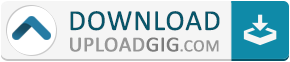#Do not remember me!

### Categories## Fx Calculator 570 991 - Solve Math by Camera 84 v4.3.4 FinalRequirements: Android 4.1+ | Size: 15,7 MB

Features:
* Basic Calculator: calculate from basic mathematics functions with percentage to advanced functions.
* Scientific Calculator: multi-functional scientific calculator with a lot of functions, all in one app.
* Equation Solver: Solve quadratic, cubic, quartic and other complex math equations.
* Camera Math: Enter math expression by using camera get answer.
* Calculation History: save your calculation history.
* Base-N calculation for programmer
* Integral calculator provide powerful calculator to calculate integrate, derivative

MATH NATURAL DISPLAY:
* See math expressions, symbols and fractions exactly the way they appear in textbooks.

SCIENTIFIC CALCULATOR:
* This Scientific Calculator contains all the scientific calculations such as equation solver, derivative, anti-trigonometric calculation, trigonometric calculation, integral, logarithmic calculation, square root calculation, power calculation, factorial calculation, cube root calculation, π calculation and so on.

PHOTO CALCULATOR - SCAN MATH PROBLEMS AND SOLVE BY CAMERA:
* Photo calculator is the most creative feature of this calculator. This feature solves problem by taking photo, inputs quickly to solve math with your camera. Unlike the other calculator, this calculator help you solve math problem quickly by taking a picture and then you can get the solutions at once.

Real simulator: calculator layout same as calculator 570 es plus and 991 fx es plus as well as 580 ex plus. It also supports most of features of ti 34, ti 36X Pro, ti 30Xa, texas & instruments calculator ti30, ti 84 plus, ti 83 Plus, ti 89. Wabbit calculator & wabbitemu calculator, grafncalc83, graphncalc83

GRAPHING - SIMULATES FOR GRAPHING CALCULATOR TI 83 AND TI 84:
* This calculator can draw simultaneously graph, this is the best way to visual functions

UNIT CONVERSION:
* A large collection of unit conversion, include real time currency converter and a lot of units such as time, length, area, ...

CALCULATION FORMULAS:
* Contains Math formulas and Physics formulas, very efficient for students in high school or university.

PROGRAMMING - THE PROGRAMMABLE CALCULATOR:
* Mathematica language is also supported by this programming calculatorWHAT'S NEW:
* Quick tile on status bar
* Graph simultaneous Descriptionting
* Graph independent zoom
* Statistical functions and documentation
* Distribution calculation
* Bug fixes and stability improvement

Homepage

Full version